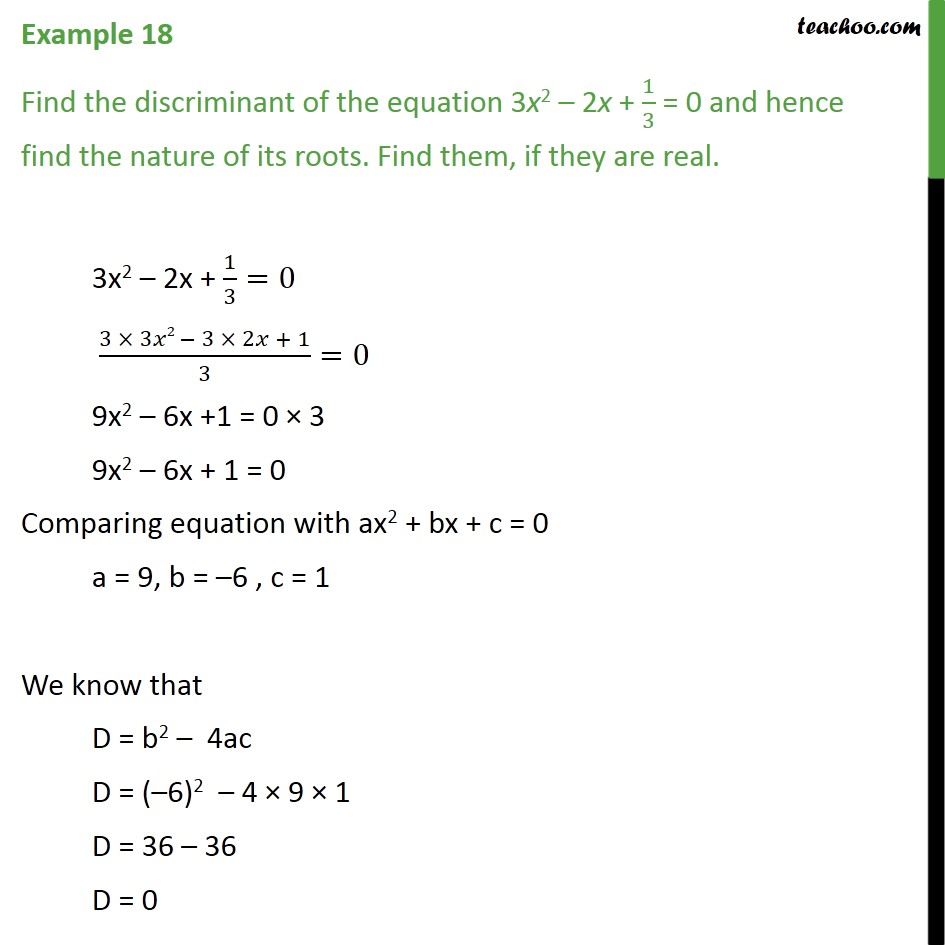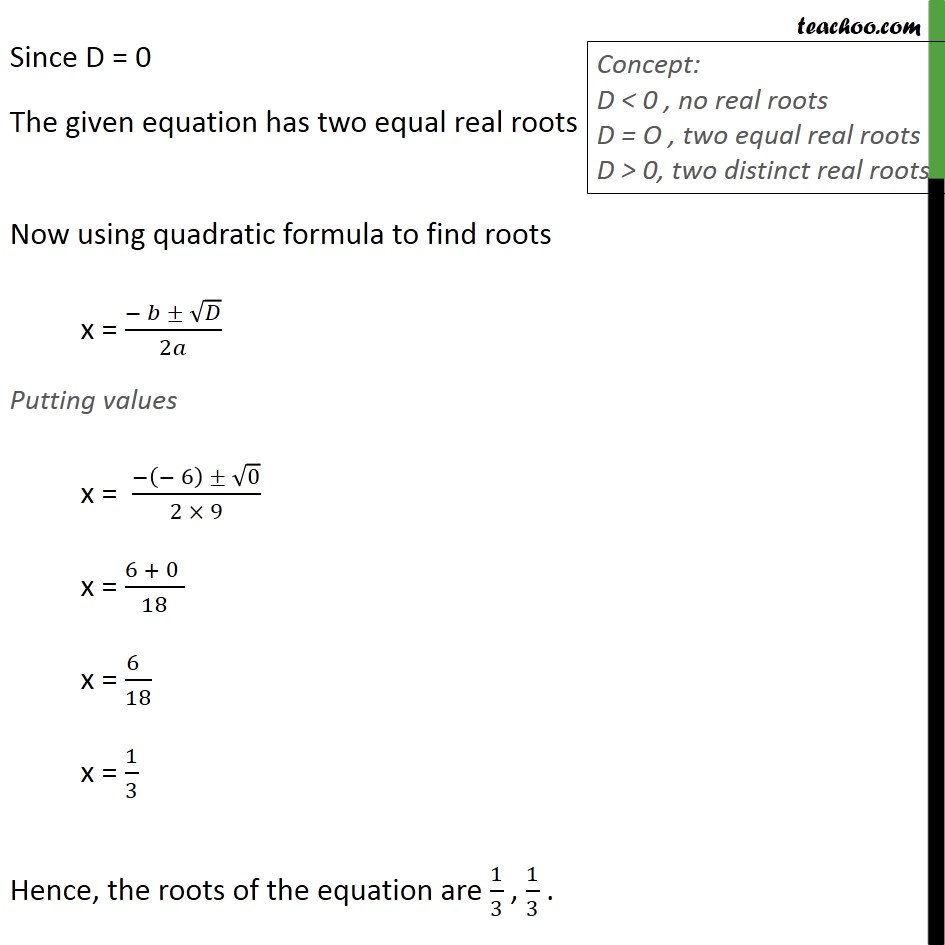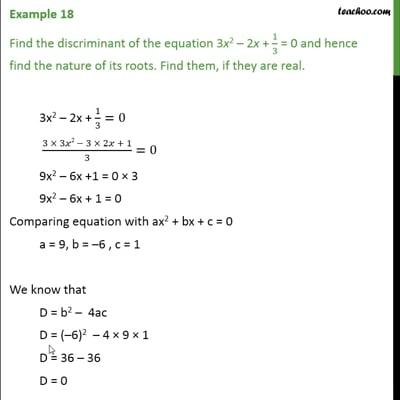Chapter 4 Class 10 Quadratic Equations

Class 10
Important Questions for Exam - Class 10This video is only available for Teachoo black users

Introducing your new favourite teacher - Teachoo Black, at only ₹83 per month

### Transcript

Example 18 Find the discriminant of the equation 3x2 2x + 1/3 = 0 and hence find the nature of its roots. Find them, if they are real. 3x2 2x + 1/3=0 (3 3 2 3 2 + 1)/3=0 9x2 6x +1 = 0 3 9x2 6x + 1 = 0 Comparing equation with ax2 + bx + c = 0 a = 9, b = 6 , c = 1 We know that D = b2 4ac D = ( 6)2 4 9 1 D = 36 36 D = 0 Since D = 0 The given equation has two equal real roots Now using quadratic formula to find roots x = ( )/2 Putting values x = ( ( 6) 0)/(2 9) x = (6 + 0 )/18 x = (6 )/18 x = 1/3 Hence, the roots of the equation are 1/3 , 1/3 .